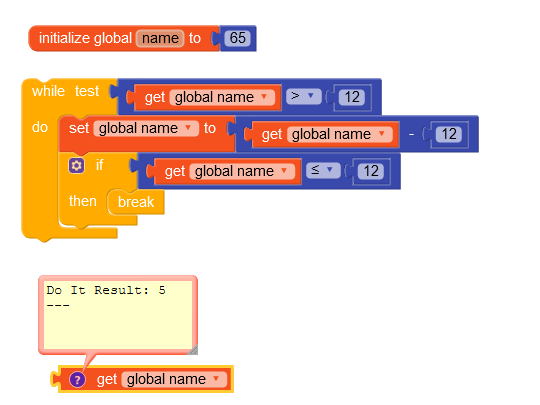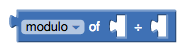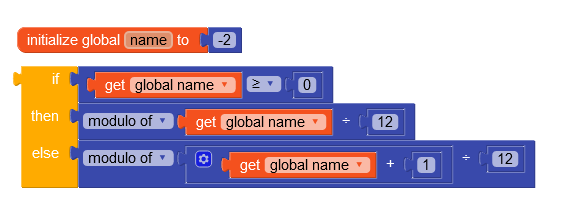# Error Select list item: Attempt to get item number 14 of a list of length 12

I have a problem. I have created a list of the 12 musical notes and I need that when I speak a number the list gives me the note for that number in the list. So far so good! The problem is when the number I ask for is larger than the list, for example, if I ask for the note with number 14 on the list, as the list has only 12 items, I want it to continue counting when it reaches the end. from the beginning, giving me the note for number 2.

Examples:

20 == 8
120 == 12
-1 == 12
-26 == -2 == 11

Does anyone know how to do this on kodular blocks?

Maybe you use a “if then else” block.

Like: if input number is equal or lower than 12
Then: select number index=input number
Else: if input number is equal or lower than 24 and greater than or equal to 13
Then: select number index=(input number-12)
Else: show error: index to big

Do you know how I can encompass all numbers above 12? And the numbers below 0 too, if possible …

Why would someone ask for a number lower than 0 ? Also, all number lower than 0 are negative.

IF number >0 and number <=12 then
(do what you have to do)
ELSE
(the number is outside the range)Because if the original grade is C and the person wants to lower 1 Semi-tone, he will get -1 and I have to make this -1 start in reverse, that is, he has to leave C and go to B, which would be the last list item.

Thanks!!!

1 Like

Can you do that? I need that when I look for item -1 it understands that it is the last item on the list, because it is the first item backwards. And so on and on.

the solution from @Boban seems to work fine…
however there might be other solutions, which use less blocks…
tip: use the modulo, remainder or quotient block…http://appinventor.mit.edu/explore/ai2/support/blocks/math#modulo
Taifun
PS: probably another daily challenge type of question?

5 Likes

This just proves that I’m bad at mathCorrected blocks5 Likes

Thanks!!!

This topic was automatically closed 30 days after the last reply. New replies are no longer allowed.